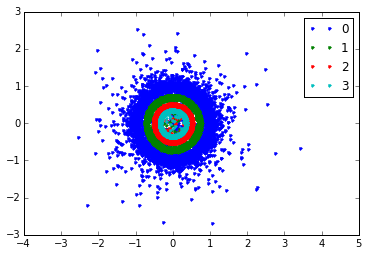## Exercise for the course Python for MATLAB users, by Olivier Verdier¶

In :
%pylab
%matplotlib inline

Using matplotlib backend: MacOSX
Populating the interactive namespace from numpy and matplotlib


Check out the formula for a companion matrix on wikipedia. Define a function companion which accepts a vector in argument, and returns the corresponding companion matrix. You can use the command diag for that. The resulting matrix should be of complex type.

In :
diag?

In [ ]:
ones?

In [ ]:
concatenate?

In :
def companion(coefficients):
size = len(coefficients)
M = diag(ones(size-1, dtype=complex), k=-1)
M[:,-1] = -coefficients
return M

In :
C = companion(ones(3))
assert(C.dtype == complex)
assert(len(C) == 3)


Fix a given size, say size = 20, and create a vector of length 20 with random, normally distributed, complex numbers. Use the randn function for that, and combine two random real vectors to get a random complex vector.

In [ ]:
randn?


Now, fix a standard deviation, say sigma = 1./10, and use the random complex coefficients obtained above, multiplied by sigma, in the companion function. Use eigvals to compute the eigenvalues, and plot them on the complex plane. You can use the command axis('equal') to make sure the plot has the same dimensions in x and y.

Finally, repeat that, say 200 times. Plot all the eigenvalues on the same figure. What do you observe? What happens when you change the standard deviation sigma?

In :
size = 20
for k in range(4):
sigma = 10**(-3*k)
es = []
for i in range(200):
coeffs = sigma*(randn(size) + 1j*randn(size))
M = companion(coeffs)
es.append(eigvals(M))
aes = array(es).reshape(-1)
plot(aes.real, aes.imag, '.',label=k)
axis('equal')
legend()

Out:
<matplotlib.legend.Legend at 0x1068e3c50>In [ ]: